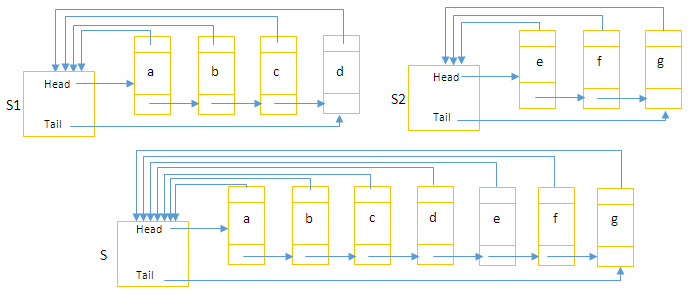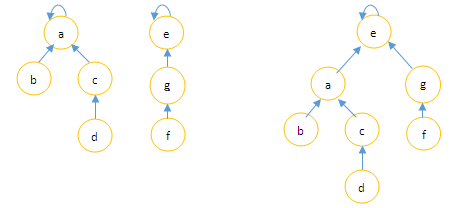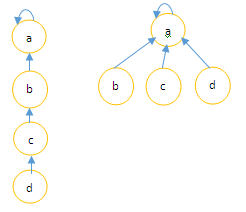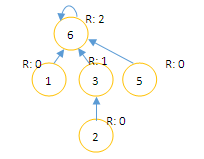# Introduction and Implementation of Disjoint Sets using c#

 Category: Data Structure And Algorithms Tags:Disjoint Sets Code Files

## Introduction

A disjoint set is a collection of elements like S = {S1, S2 … Sk}. We identify set with a representative which can be anything like first element of set or lowest element etc. We have three operations on sets:
MakeSet(x): Creates set with one element x
Union(x, y): Union two sets x and y
FindSet(x): Finds representative of a set using x (an element of set)

## Implementation

Linked List Representation:- linked list representation is a linear representation of set, where each set object has head and tail and each element’s next pointer points next element and other pointer points back to set itself. Head of set is it's representative.Fig: Linked list representation of sets

As we can see set above, we can easily union two sets by making next pointer of "d” to other set’s head, all head pointers of other set to first set’s object and first set’s tail pointer to second set’s last element.

Still it won’t give us optimistic running time, for that we can use tree structure to make sets where root will be representative and all other nodes will be pointing to parent and we can implement heuristics to improve running time, below tree structure of sets are given:Fig: Tree representation of sets

Above we can see we have tree structure of sets and if we want to union two we have to find their representative and then merge, we have our first heuristic Union by Rank where we assign rank to every node of tree based on it’s height in tree and while union operation we compare ranks of representatives of both trees, the one with higher rank will be parent of other one, if both has same rank we just can chose arbitrarily any tree to be parent of other. Union by Rank applies on union operation other heuristic we have shown in FindSet method is Path Compression. Path compression makes all nodes of tree pointing directly to root of the tree and will not change any ranks.Fig: Path Compression

Let’s see implementation of MakeSet, Union and FindSet. Here we are going to use arrays to maintain rank and parent nodes. Let’s suppose there are natural numbers 0,1,2,3,4…n and an array "parent" indexed from 0 to n which index represents naturals numbers and value at index is index of parent node. Other array "rank" represent rank of a node drawn from index value.

```public class Set
{
int[] parent;   // stores index of parent node
int[] rank;     //stores rank of perticular node    /// <summary>
/// Initialize n natural numbers
/// </summary>
/// <param name="length">value of n</param>
public Set(int length)
{
parent = new int[length];   //initializng length of set
rank = new int[length];
//initially making parent of element itself
for (int i = 0; i < parent.Length; i++)
parent[i] = i;
}
public void MakeSet(int x){...}
public void Union(int x, int y){...}
public int FindSet(int x) {...}    public int FindImmidiateParent(int x)    public int FindRank(int x)
}
```

Above given structure of class "Set" is having two arrays parent and rank and constructor which initializes arrays based on length and initially makes parent of each element is element itself. Below we will see implementation of MakeSet method:

```/// <summary>
/// Making set with one element
/// </summary>
/// <param name="x">element</param>
public void MakeSet(int x)
{
parent[x] = x;  //making parent itself
rank[x] = 0;    //initially rank is zero
}
```

MakeSet method creates sets with only one element and representative to element itself (parent). Now we can create bigger sets by Union of two sets:

```/// <summary>
/// Union by rank
/// </summary>
/// <param name="x">set 1</param>
/// <param name="y">set 2</param>
public void Union(int x, int y)
{
int representativeX = FindSet(x); //finding representative of x
int representativeY = FindSet(y); //finding representative of y
//if both has same rank maiking y is x's parent
if (rank[representativeX] == rank[representativeY])
{
rank[representativeY]++; //incrementing rank of y
parent[representativeX] = representativeY;
}
// making parent which is having higher rank
else if (rank[representativeX] > rank[representativeY])
{ parent[representativeY] = representativeX; }
else
{ parent[representativeX] = representativeY; }
}
```

Above Union works on heuristic Union by Rank where rank will be compared and higher rank set will be parent of other. Union calls FindSet method which implementation is:

```/// <summary>
/// Finds representative of a set
/// </summary>
/// <param name="x">element of a set</param>
/// <returns></returns>
public int FindSet(int x)
{
if (parent[x] != x)
parent[x] = FindSet(parent[x]); //path compression
return parent[x];
}
```

The implementation of rest of the methods is:

```/// <summary>
/// Finding Immidiate Parent
/// </summary>
/// <param name="x"></param>
/// <returns></returns>
public int FindImmidiateParent(int x)
{
return parent[x];
}
/// <summary>
/// Finding Rank
/// </summary>
/// <param name="x"></param>
/// <returns></returns>
public int FindRank(int x)
{
return rank[x];
}```

FindSet uses heuristic Path Compression which makes all nodes pointing directly to root. Now we can see how it works:

```static void Main(string[] args)
{
//vertices
int[] vertices = new int[] { 1, 2, 3, 5, 6 };
//just a bigger number than highest value of vertices
Set set = new Set(100);
foreach (int vertex in vertices)
{
set.MakeSet(vertex);
}
//unions
set.Union(2, 3);
set.Union(5, 6);
set.Union(3, 6);
set.Union(6, 1);
//printing final set
foreach (int vertex in vertices)
{
Console.WriteLine("parent of {0} is: {1} and rank is: {2}", vertex, set.FindImmidiateParent(vertex), set.FindRank(vertex));
}

}
```

Above we can see first we created five sets with elements 1,2,3,5,6 then we performed union on {2,3}, {5,6}, {3,6},{6,1} which will create tree forest where 3 is parent of 2, 6 is parent of 5, 6 is parent of 3 and 6 is parent of 1(here rank of 6 is greater than rank of 1 so 6 will be parent of 1).

Output:

parent of 1 is: 6 and rank is: 0

parent of 2 is: 3 and rank is: 0

parent of 3 is: 6 and rank is: 1

parent of 5 is: 6 and rank is: 0

parent of 6 is: 6 and rank is: 2

We can create tree based on values of parent and rank:Fig: Set tree after union

Above in figure you can see final tree after MakeSet and Union and we can see ranks where rank of 6 is highest.

 Like0 PeopleNikhil Joshi Ceo & Founder at Dotnetlovers Atricles: 146 Questions: 16 Given Best Solutions: 16 *

Login via:x